# Near-ring

(diff) ← Older revision | Latest revision (diff) | Newer revision → (diff)

One of the generalizations of the concept of an associative ring (cf. Associative rings and algebras). A near-ring is a ringoid over a group, i.e. a universal algebra in which an associative multiplication and addition exist; a near-ring is a (not necessarily Abelian) group with respect to addition, and the right distributive property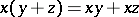must hold too. A near-ring is also an example of a multi-operator group.

Examples of near-rings are the set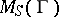of all mappings of a groupinto itself which commute with the action of a given semi-groupof endomorphisms of. The group operations inare defined pointwise and multiplication inis composition of mappings. A near-ringis an analogue of a ring of matrices. The notions of a sub-near-ring, of an ideal and of a right module over a near-ring are introduced in the usual manner.

Let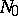() be the variety of near-rings defined by the identity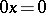(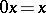). Every near-ringcan be decomposed into the sum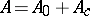of sub-near-rings, where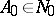,and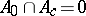. A cyclic right-moduleis called primitive of typeifis simple; primitive of type 1 if either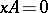or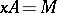for any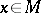; and primitive of type 2 ifis a simple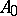-module. A near-ringis called primitive of type(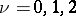) if there is a faithful simple-moduleof type. In this case there is a dense imbedding ofintofor some semi-groupof endomorphisms of. For-primitive near-ringswith an identity element and with the minimum condition for right ideals in, the equality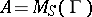holds (an analogue of the Wedderburn–Artin theorem). For every, the Jacobson radicalof typecan be introduced as the intersection of the annihilators of-primitive-modules. The radicalis defined as the intersection of the maximal right module ideals. All four radicals are different, and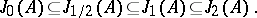It turns out that these radicals posses many properties of the Jacobson radical of an associative ring (cf. ).

For near-rings an analogue of Ore's theorem on near-rings of fractions  holds.

A distributively-generated near-ring is a near-ring whose additive group is generated by elementssuch that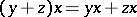for allandin the near-ring. All distributively-generated near-rings generate the variety. For finite distributively-generated near-rings the notions of 1- and-primitivity coincide;-primitive distributively-generated near-rings have the formfor some group. In a distributively-generated near-ring with the identitymultiplication is commutative (cf. , ).

Every near-ring fromwithout nilpotent elements is a subdirect product of near-rings without divisors of zero . A near-algebracan be decomposed into a direct sum of simple near-rings if and only if: a) it satisfies the minimum condition for principal ideals; b)does not contain ideals with zero multiplication; and c) any annihilator of any minimal ideal is maximal .

For near-rings one can prove results similar to those on the structure of regular rings  and on near-rings of fractions . Near-rings have applications in the study of permutation groups, block-schemes and projective geometry .

How to Cite This Entry:
Near-ring. Encyclopedia of Mathematics. URL: http://encyclopediaofmath.org/index.php?title=Near-ring&oldid=15999
This article was adapted from an original article by V.A. Artamonov (originator), which appeared in Encyclopedia of Mathematics - ISBN 1402006098. See original article